# Simultaneous Equations

## Activities

Simultaneous Shopping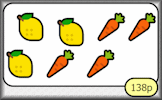Find the cost of the individual items in shopping baskets (introduction to simultaneous equations).

Algebra In Action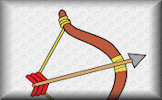Real life problems adapted from an old Mathematics textbook which can be solved using algebra.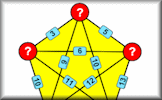Find the five numbers which when added or multiplied together in pairs to produce the given sums or products.

Recurring Decimals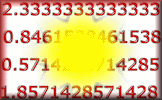Change recurring decimals into their corresponding fractions and vica versa.

Simultaneous Equations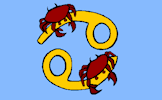A self-marking, multi-level set of exercises on solving pairs of simultaneous equations.

Simultaneous Solutions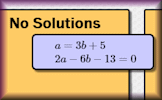Arrange the given pairs of simultaneous equations in groups to show whether they have no solution, one solution or infinite solutions.

Unbeknownst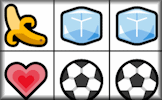Some picture grid puzzles which can be solved by using simultaneous equations.

Using GraphsUse the graphs provided and create your own to solve both simultaneous and quadratic equations.

Window View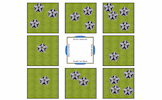Drag the 20 flowers into the gardens so that 9 flowers are visible from each window of the house.

## Investigations

Window ViewDrag the 20 flowers into the gardens so that 9 flowers are visible from each window of the house.

## Videos

This is the main Transum help video on Simultaneous Equations.

Simultaneous Equations Video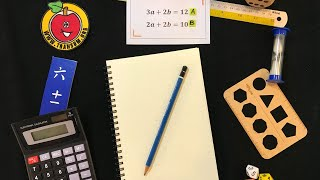Learn to solve simultaneous equations from the very basic to those containing quadratics. This video is to help you do the online, self-marking exercise.

Simultaneous Equations (Elimination)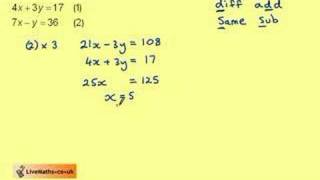This video demonstrates how to solve simultaneous equations by elimination.

Simultaneous Graphs Video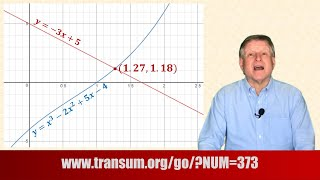A short video which explains how to use graphs to solve simultaneous equations.

## StartersBoxing Day
Work out the contents and the cost of the Christmas boxes from the given clues.Light Shopping
A lamp and a bulb together cost 32 pounds. The lamp costs 30 pounds more than the bulb. How much does the bulb cost?Oblongs
Find the dimensions of a rectangle given the perimeter and area.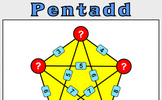Five numbers are added together in pairs and the sums shown. What might the five numbers be?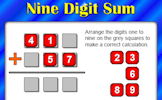Planet Numpair
The sum and product are given, can you find the two numbers?Rabbits and Chickens
There are some rabbits and chickens in a field. Calculate how many of each given the number of heads and feet.Rail Weigh
Use the weights of the trains to work out the weight of a locomotive and a coach. A real situation which produces simultaneous equations.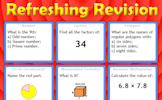Refreshing Revision
It is called Refreshing Revision because every time you refresh the page you get different revision questions.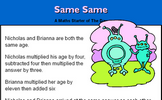Same Same
A problem involving two people's ages which can be solved using algebra.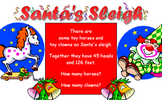Santa's Sleigh
Work out the number of clowns and horses given the number of heads and feet.Simultaneous Occasions
A problem which can best be solved as a pair of simultaneous equations.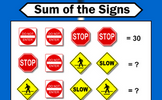Sum of the Signs
Each traffic sign stands for a number. Some of the sums of rows and columns are shown. What numbers might the signs stand for?Summer Holidays
How many children and how many donkeys are on the beach? You can work it out from the number of heads and the number of feet!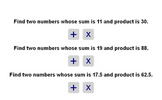Two Numbers
Find the two numbers whose sum and product are given.

27 items are currently in this category.

Teachers might find the complete Simultaneous Equations Topic List useful.

This topic covers simultaneous equations with two different variables. The starters pose real world problems which can be solved using the techniques taught at school or by other intuitive methods.

Though there are many formal strategies for solving simultaneous equations the skill of forming the equations from real life situations is a very important stage in working towards a solution.

Algebraic methods are the most efficient for solving basic simultaneous equations but graphical methods, probably using a graphic display calculator or computer software package, may be more suitable for less standard sets of simultaneous equations.For All: